## Polynomial Time Approximation Scheme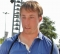Updated on 17-Jun-2020 10:07:44
Polynomial Time Approximation schemeWe can find some polynomial time solution for NP-Complete problems like 0-1 Knapsack problem or Subset sum problem. These problems are very popular in the real world, so there must be some ways to handle these problems.The Polynomial Time Approximation Scheme (PTAS) is a type to approximate algorithms for optimization problems. For the 0-1 Knapsack problem, there is a Pseudo Polynomial Solution, but when the values are large, the solution is not feasible. Then we need a PTAS solution.Some NP-complete problems like Graph Coloring, K-Center problem etc. they have no known polynomial time solution. PTAS used to approximate ... Read More

## What is 'Space Complexity’?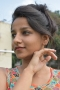Updated on 17-Jun-2020 10:08:53
Space ComplexitySpace complexity is an amount of memory used by the algorithm (including the input values of the algorithm), to execute it completely and produce the result.We know that to execute an algorithm it must be loaded in the main memory. The memory can be used in different forms:Variables (This includes the constant values and temporary values)Program InstructionExecutionAuxiliary SpaceAuxiliary space is extra space or temporary space used by the algorithms during its execution.Memory Usage during program executionInstruction Space is used to save compiled instruction in the memory.Environmental Stack is used to storing the addresses while a module calls another module ... Read More

## Amortized Analysis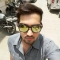Updated on 17-Jun-2020 10:07:00
Amortize AnalysisThis analysis is used when the occasional operation is very slow, but most of the operations which are executing very frequently are faster. Data structures we need amortized analysis for Hash Tables, Disjoint Sets etc.In the Hash-table, the most of the time the searching time complexity is O(1), but sometimes it executes O(n) operations. When we want to search or insert an element in a hash table for most of the cases it is constant time taking the task, but when a collision occurs, it needs O(n) times operations for collision resolution.Aggregate MethodThe aggregate method is used to find ... Read More

## Asymptotic NotationsUpdated on 17-Jun-2020 10:09:54
Asymptotic NotationsAsymptotic notations are used to represent the complexities of algorithms for asymptotic analysis. These notations are mathematical tools to represent the complexities. There are three notations that are commonly used.Big Oh NotationBig-Oh (O) notation gives an upper bound for a function f(n) to within a constant factor.We write f(n) = O(g(n)), If there are positive constantsn0  and c such that, to the right of n0 the f(n) always lies on or below c*g(n).O(g(n)) = { f(n) : There exist positive constant c and n0 such that 0 ≤ f(n) ≤ c g(n), for all n ≥ n0}Big Omega NotationBig-Omega ... Read More

## Asymptotic Analysis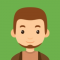Updated on 17-Jun-2020 10:11:12
Asymptotic AnalysisUsing asymptotic analysis, we can get an idea about the performance of the algorithm based on the input size. We should not calculate the exact running time, but we should find the relation between the running time and the input size. We should follow the running time when the size of the input is increased.For the space complexity, our goal is to get the relation or function that how much space in the main memory is occupied to complete the algorithm.Asymptotic BehaviorFor a function f(n) the asymptotic behavior is the growth of f(n) as n gets large. Small input ... Read More

## Algorithms and ComplexitiesUpdated on 17-Jun-2020 10:12:43
AlgorithmAn algorithm is a finite set of instructions, those if followed, accomplishes a particular task. It is not language specific, we can use any language and symbols to represent instructions.The criteria of an algorithmInput: Zero or more inputs are externally supplied to the algorithm.Output: At least one output is produced by an algorithm.Definiteness: Each instruction is clear and unambiguous.Finiteness: In an algorithm, it will be terminated after a finite number of steps for all different cases.Effectiveness: Each instruction must be very basic, so the purpose of those instructions must be very clear to us.Analysis of algorithmsAlgorithm analysis is an important part ... Read More

## Operating system time slicing in round robin scheduling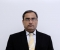Updated on 20-Jun-2020 09:50:34
process Burst time A 4 B 1 C 8 D 1time slice=10 unitA B C D A C C C 0 2 3 5 6 8 10 12 14So A will complete 8 cycles.